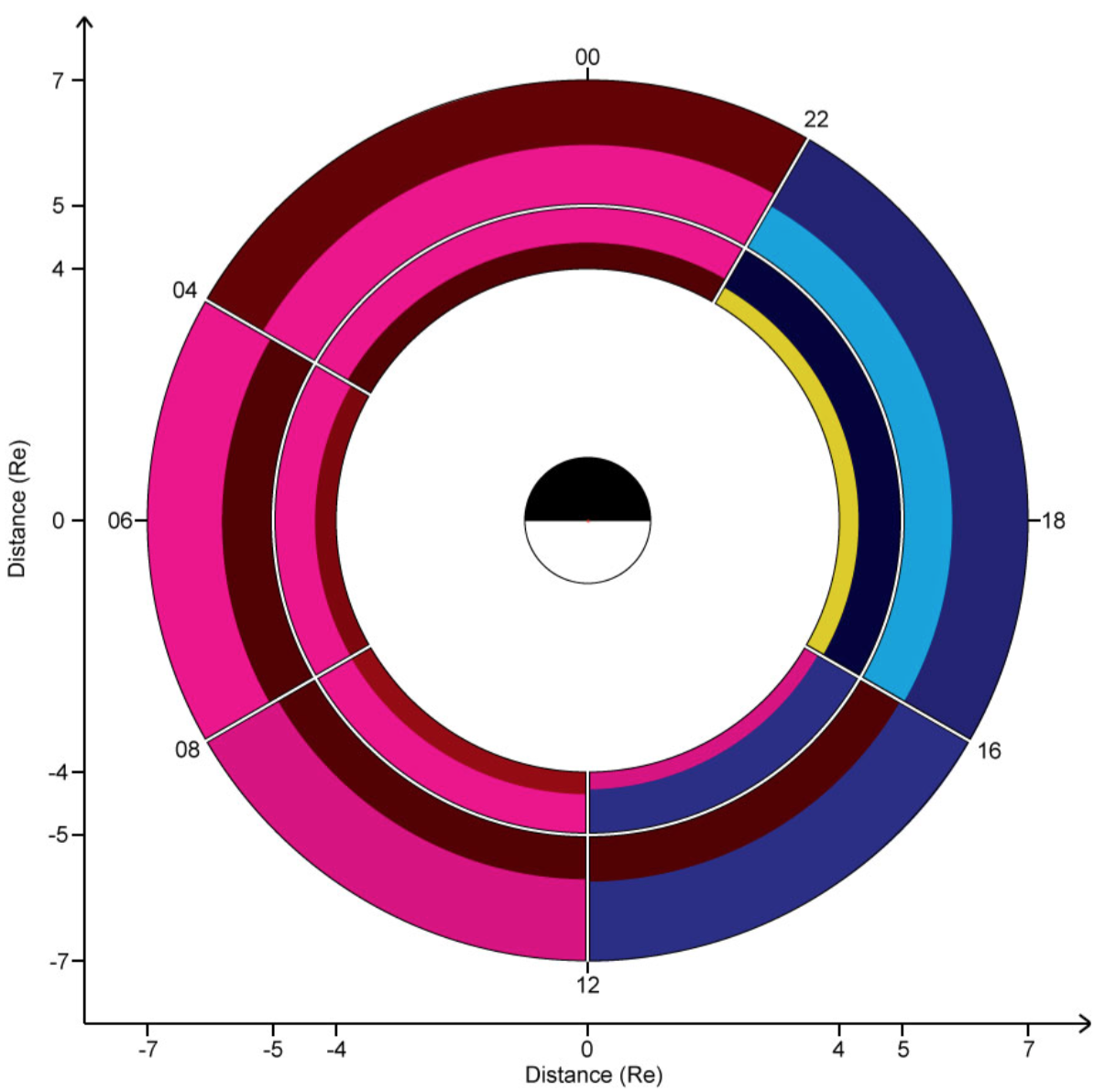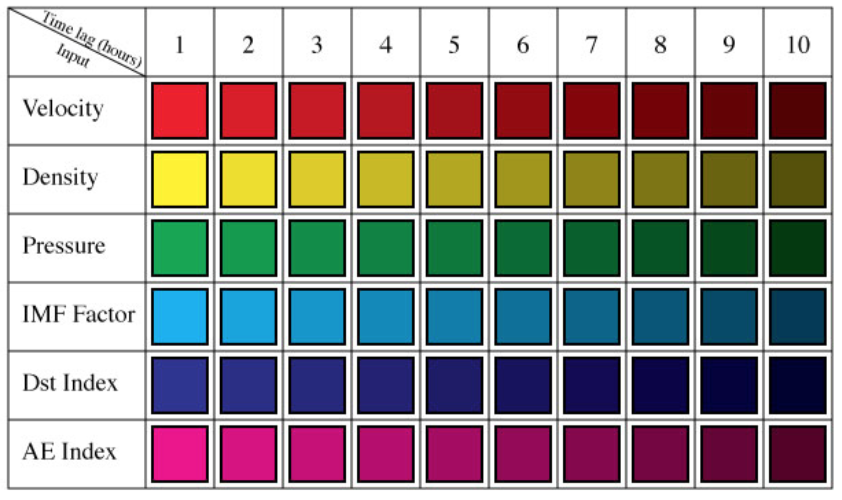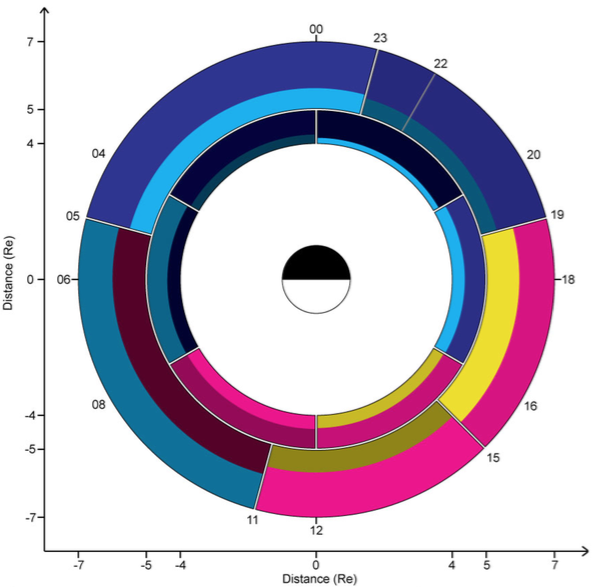## Statistical Wave Models

Magnetic field wave data from the Cluster and THEMIS missions has been used as the basis to compute new statistical wave models for the amplitudes of lower band chorus, hiss, and equatorial magnetosonic waves. The models are based on the results of an Error Reduction Ratio analysis of solar wind parameters, location, and geomagnetic indices in order to determine which of the input set of parameters are in most influential of the evolution of the magnetic field amplitudes of the wave modes under consideration.

The plots below show which are the two most important parameters in the evolution of the wave activity of a particular wave mode. Clicking on a particular sector will display the coefficients of the statistical wave model for that sector.

### Lower band chorusNotes: None

### HissNotes: For both L-shell ranges there is an overlap in MLT such that the models are actually calculated for MLT ranges 20 < MLT < 00 and 22 < MLT < 04.Notes: For L-shell range 5 < L < 7 there is an overlap in MLT such that the models are actually calculated for MLT ranges 19 < MLT < 23 and 22 < MLT < 05.